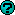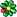Wrox Programmer ForumsConverting int to string
 |
 C++ Programming General discussions for the C++ language. For questions specific to Microsoft's Visual C++ variant, see the Visual C++ forum instead.
 Welcome to the p2p.wrox.com Forums. You are currently viewing the C++ Programming section of the Wrox Programmer to Programmer discussions. This is a community of software programmers and website developers including Wrox book authors and readers. New member registration was closed in 2019. New posts were shut off and the site was archived into this static format as of October 1, 2020. If you require technical support for a Wrox book please contact http://hub.wiley.com
 JudexRegistered User Join Date: Aug 2003 Posts: 7 Thanks: 0 Thanked 0 Times in 0 PostsConverting int to string

I am writing a lexical analyser in C. I need to know how to convert integer to string . The other way round I can do it.

Thanks

judexGertAuthorized User Join Date: Jun 2003 Posts: 95 Thanks: 0 Thanked 0 Times in 0 PostsHi. I use the following code to do this in Visual C++. Maybe you can use it. I am sure there are better ways to do this, but it works for my purposes. (It is not my code, but you may use it...)

Code:
```CString CYourClass::IntToString(int number)
{
int i=0;
int antdes=0;    // = number of digits
CString temp;
BOOL negsign=FALSE;

if(number<0)
{
negsign=TRUE;
number=abs(number);
}

temp.Empty();
if(number>9999999999999999999)
antdes=20;
else if(number>999999999999999999)
antdes=19;
else if(number>99999999999999999)
antdes=18;
else if(number>9999999999999999)
antdes=17;
else if(number>999999999999999)
antdes=16;
else if(number>99999999999999)
antdes=15;
else if(number>9999999999999)
antdes=14;
else if(number>999999999999)
antdes=13;
else if(number>99999999999)
antdes=12;
else if(number>9999999999)
antdes=11;
else if(number>999999999)
antdes=10;
else if(number>99999999)
antdes=9;
else if(number>9999999)
antdes=8;
else if(number>999999)
antdes=7;
else if(number>99999)
antdes=6;
else if(number>9999)
antdes=5;
else if(number>999)
antdes=4;
else if(number>99)
antdes=3;
else if(number>9)
antdes=2;
else
antdes=1;
for(i=0;i<antdes;i++)
{
temp+=(char)((number%10)+48);
number/=10;
}
if(negsign)
temp+="-";
temp.MakeReverse();
return temp;
}```
Gert
 davekw7xAuthorized User Join Date: Feb 2004 Posts: 76 Thanks: 0 Thanked 0 Times in 0 PostsIf you want to implement a function to convert an integer to a string,
there are lots of ways. Is this what you want to do?

If your assignment is to write a lexical analyser in C, then I
would assume you can use standard C functions, such as sprintf(),
which is declared in <stdio.h>.

Look it up.

Dave

Quote:
 quote:Originally posted by Judex   I am writing a lexical analyser in C. I need to know how to convert integer to string . The other way round I can do it. Thanks judextfalcaoRegistered User Join Date: Mar 2004 Posts: 3 Thanks: 0 Thanked 0 Times in 0 PostsThere are lots of ways...
Example in C:
value: Number to be converted
string: String result
radix: Base of value, must be in the range 2 â 36

Quote:
 quote:Originally posted by Judex   I am writing a lexical analyser in C. I need to know how to convert integer to string . The other way round I can do it. Thanks judexThiago FalcÃ£oSimilar Threads Thread Thread Starter Forum Replies Last Post Converting int to byte yukijocelyn C# 2005 1 May 30th, 2008 03:44 AM Error converting data type varchar to int. - SQL baysaa SQL Server ASP 1 March 16th, 2008 11:56 PM Converting Int -> ASCII silaros C++ Programming 1 June 5th, 2007 01:12 AM MySQL return a String instead of an INT peg110 PHP Databases 2 December 2nd, 2004 01:23 PM Converting String to an integer Louisa VB.NET 2002/2003 Basics 2 March 5th, 2004 10:25 AM